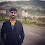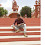### Maximum Absolute Difference - The Coding Shala

Home >> Programming Questions >> Maximum Absolute Difference

## Maximum Absolute Difference InterviewBit Solution

You are given an array of N integers, A1, A2,…, AN. Return maximum value of `f(i, j)` for all 1 ≤ i, j ≤ N.
`f(i, j)` is defined as `|A[i] - A[j]| + |i - j|`, where |x| denotes the absolute value of x.

For example,
```
A=[1, 3, -1]
```
f(1, 1) = f(2, 2) = f(3, 3) = 0

f(1, 2) = f(2, 1) = |1 - 3| + |1 - 2| = 3

f(1, 3) = f(3, 1) = |1 - (-1)| + |1 - 3| = 4
f(2, 3) = f(3, 2) = |3 - (-1)| + |2 - 3| = 5

So, we return 5.
``````

#### Solution

Here function f(i, j) = |A[i] - A[j]| + |i-j] can be written in four ways -

``````(A[i] + i) - (A[j] + j)
-(A[i] - i) + (A[j] - j)
(A[i] - i) - (A[j] - j)
(-A[i] - i) + (A[j] + j) = -(A[i] + i) + (A[j] + j)``````
we just have to store minimum and maximum values of expressions `A[i] + i` and `A[i] - i` for all `i.`

Java Code::
 ``` 1 2 3 4 5 6 7 8 9 10 11 12 13 14 15``` ```public class Solution { public int maxArr(ArrayList A) { int max1 = Integer.MIN_VALUE; int max2 = Integer.MIN_VALUE; int min1 = Integer.MAX_VALUE; int min2 = Integer.MAX_VALUE; for(int i=0;i

Other Posts You May Like

1.This question can be solved in O(N) Time and O(1) space complexity by using simple mathematics concepts of absolute operator.

We can deduce this express into 4 different forms after removing the absolute operator and applying specific conditions.

Cases can be A[i]>A[j] or A[i]<=A[j] and simultaneously i>j and i<=j can be the cases so using these conditions we can formulate 4 different expressions which do not involve use of absolute operator.
After that we just need to find the maximum value possible through each expression by iterating on array of numbers only once.

If you still face any difficulties while solving this question then you can refer to the video solution

1.Nice Explanation.

2.Code is very simple once we understand the logic. Thanks Vaibhav. Breaking down the modulus operation into all possibilities made it clear.